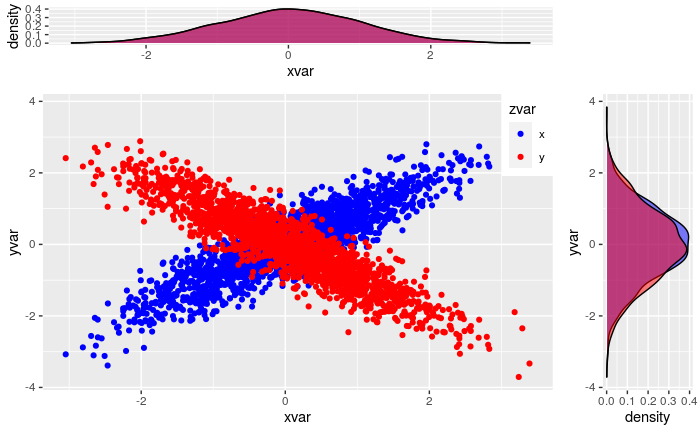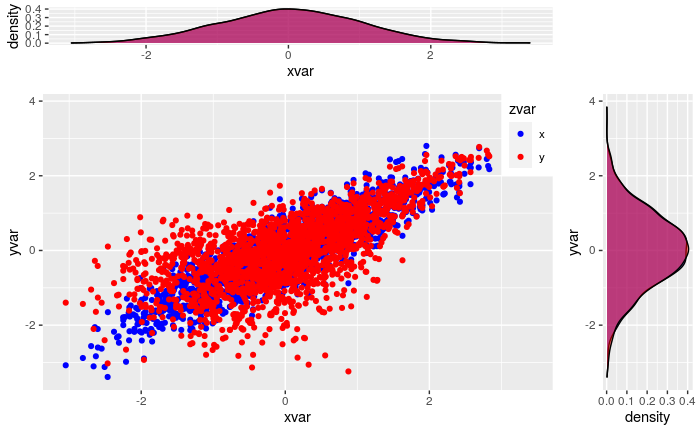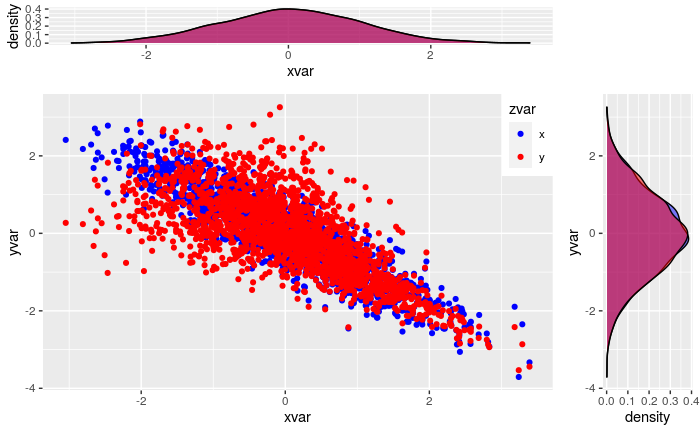Version 0.3.0 of ESGtoolkit has been released – on GitHub for now. As in v0.2.0, it contains functions for the simulation of dependent random variables. Here is how you can install the package from R console:

library(devtools)
devtools::install_github("Techtonique/esgtoolkit")


When I first created ESGtoolkit back in 2014, its name stood for Economic Scenarii Generation toolkit. But since the underlying technique is an exact simulation of – solutions of – Stochastic Differential Equations (SDE), the ESG part of the name is more or less irrelevant now. SDEs are, indeed, used to describe the dynamics of several physical systems. The main changes in v0.3.0 are:

-The use of VineCopula instead of CDVine (soon to be archived) for the simulation of dependencies between random variables. More on this later in the post, and thanks to Thomas Nagler, Thibault Vatter and Ulf Schepsmeier for the fruitful discussions.

-A new website section for the package, created with pkgdown, and including documentation + examples + loads of graphs: https://techtonique.github.io/.

It’s worth noticing that ESGtoolkit is not destined to evolve at a great pace. And notably, there’ll never be model calibration features, as those are already available in a lot of tools out there in the wild.

# But what are Copulas?

Simply put, Copulas (as in VineCopula) are functions which are used to create flexible multivariate dependencies from marginal distributions. What does that mean? What we’re more accustomed to as a dependency between variables is linear correlation. Commonly, correlation. For example, if we let Sales be your dollar sales of kombucha per month, and Advert be your advertising costs, then the linear correlation between Sales and Advert is (very) roughly, the frequency at which they both vary in the same direction, when put on the same scale.

When linear correlation is close to 1, Sales and Advert mostly vary in the same direction. Otherwise, when it’s close to -1, Sales and Advert mostly vary in opposite directions. But linear correlation is… linear. The intuition behind this, is the proportionality of the correlation coefficient with the slope of a simple linear regression model. Linearity implies, it doesn’t take into account more complex, nonlinear types of dependencies which can occur quite frequently though.

# Examples

Not familiar with R? You can skip this introductory code and report to the next section.

## Code

devtools::install_github("Techtonique/esgtoolkit")
library(ESGtoolkit)

## Simulation parameters

# Number of risk factors
d <- 2

# Number of possible combinations of the risk factors
dd <- d*(d-1)/2

# Copula family : Gaussian -----
fam1 <- rep(1,dd)
# Correlation coefficients between the risk factors (d*(d-1)/2)
par0_1 <- 0.9
par0_2 <- -0.9

# Copula family : Rotated Clayton (180 degrees) -----
fam2 <- 13
par0_3 <- 2

# Copula family : Rotated Clayton (90 degrees) -----
fam3 <- 23
par0_4 <- -2


## Simulation of the d risk factors

# number of simulations for each variable
nb <- 500

# Linear correlation = 1
s0_par1 <- simshocks(n = nb, horizon = 4,
family = fam1, par = par0_1)

# Linear correlation = -1
s0_par2 <- simshocks(n = nb, horizon = 4,
family = fam1, par = par0_2)

# Rotated Clayton Copula (180 degrees)
s0_par3 <- simshocks(n = nb, horizon = 4,
family = fam2, par = par0_3)

# Rotated Clayton Copula (90 degrees)
s0_par4 <- simshocks(n = nb, horizon = 4,
family = fam3, par = par0_4)


## Linear correlation +1 and -1

Same distribution on the marginals (Normal), different type of dependency:

• blue: correlation = +1
• red: correlation = -1
ESGtoolkit::esgplotshocks(s0_par1, s0_par2)## Correlation +1 and Rotated Clayton Copula (180 degrees)

Same distribution on the marginals (Normal), different type of dependency:

• blue: correlation = +1
• red: Rotated Clayton Copula (180 degrees)
ESGtoolkit::esgplotshocks(s0_par1, s0_par3)## Correlation -1 and Rotated Clayton Copula (90 degrees)

Same distribution on the marginals (Normal), different type of dependency:

• blue: correlation = +1
• red: Rotated Clayton Copula (90 degrees)
ESGtoolkit::esgplotshocks(s0_par2, s0_par4)When we observe rotated Clayton copula’s simulated points in the second and third graphs, we see patterns which can’t be reproduced when using linear correlation, with actual danger zones: wherever linear correlation can’t go for certain. For example, the quadrant [-4, -2] x [-2, 0] in the last graph.